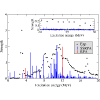Physical Review Letters ( IF 8.385 ) Pub Date : 2020-11-20 , DOI: 10.1103/physrevlett.125.212501
D. Gambacurta; M. Grasso; J. EngelWe develop a fully self-consistent subtracted second random-phase approximation for charge-exchange processes with Skyrme energy-density functionals. As a first application, we study Gamow-Teller excitations in the doubly magic nucleus ${}^{48}\mathrm{Ca}$, the lightest double-$\beta$ emitter that could be used in an experiment, and in ${}^{78}\mathrm{Ni}$, the single-beta-decay rate of which is known. The amount of Gamow-Teller strength below 20 or 30 MeV is considerably smaller than in other energy-density-functional calculations and agrees better with experiment in ${}^{48}\mathrm{Ca}$, as does the beta-decay rate in ${}^{78}\mathrm{Ni}$. These important results, obtained without ad hoc quenching factors, are due to the presence of two-particle–two-hole configurations. Their density progressively increases with excitation energy, leading to a long high-energy tail in the spectrum, a fact that may have implications for the computation of nuclear matrix elements for neutrinoless double-$\beta$ decay in the same framework.

down
wechat
bug